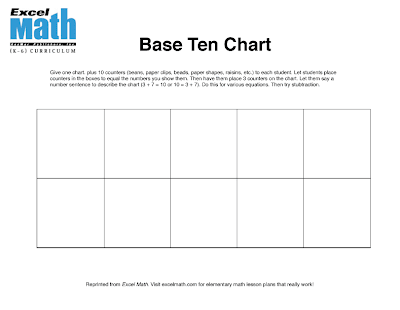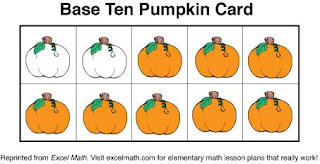## Thursday, October 25, 2012

### Base Ten Learning Tools

In Excel Math, we help students learn to add and subtract numbers using everyday objects, hands-on experiences, number cards, reproducible manipulatives and even mental math. We've created some Pumpkin Base Ten Cards you can print for the fall season. Here are some ideas you can use in your classroom.Pumpkin Base Ten Cards
Make a set of cards for each student to help them identify and add numbers. Or use them as flash cards. Cut apart the cards. Hold up a card and have students tell you the number. Then have them write the number. Remind them to write neatly. Place several cards on your whiteboard pen tray or tape them to a wall and let students put them in order from least to greatest. (Use removable tape.) Do the same with students holding the cards while another student arranges them in order. Have the students mix up, and let another student put them in order. Mix them up again and have another student arrange them in order from greatest to least.

For addition practice, give students counters (beans, pumpkin seeds, beads, paper clips, etc.). Let students add a counter to each empty space and then write the combination for the equation:
3 + 7 = 10 or 10 = 3 + 7

Show a card and have your students tell you how many more pumpkins would be needed to make ten. Check the answer by having a student count the empty boxes. (It would take 7 more.) Then have a student tell you the corresponding number sentence:
3 plus 7 make 10, or 3 + 7 = 10 or 10 = 3 + 7

Have students shuffle their cards. Let each student take one card from her pile. Have the students add the pumpkins to find the total. Then have them create a word problem for that number sentence.

To help your students understand the visual patterns of numbers and their relationships, here is a blank base 10 chart you can download and use with your class. Give each child a chart plus 10 counters. You can use beads, paper clips, raisins, dried beans, small blocks, paper shapes or counters of your choice. Draw a number on the board. Let students place counters in the boxes to equal the numbers you show them. Point out that one full row equals 5.Base Ten Chart Click on this link to download the PDf file.
For addition practice, write the number 3 on the board. Have your students place three counters on the chart. Ask them how many more counters they would need to add to the chart to make 10. Let them say a number sentence to describe that equation (3 + 7 = 10 or 10 = 3 + 7). Do this for various equations. When your students are comfortable with addition, try subtraction.

For your Kindergarten students, give each child a set of number cards from the Excel Math Student Lesson Sheets or from the Manipulatives in the Teacher Edition. Hold up a Pumpkin Card and let the student find the matching number card. Older students can match the Pumpkin Cards with their appropriate number words.Excel Math Kindergarten Number Cards from Lesson 15
Use the pumpkin base ten cards for subtraction. Let your students create story problems to go with each card. For this equation: 10 - 7 = 3 you could create the following story:
Jamie had 10 pumpkins. He gave 7 to his friends and neighbors. Now he has three left.Excel Math Pumpkin Card from the PDF link above
Use the cards with white and orange pumpkins for additional addition and subtraction practice. Combine cards to add larger numbers. Have children work in pairs to add and subtract using the cards and a timer. See how many cards each student can add in one minute. Play again and see how many students can beat their original time.

Play a card game having children turn over two cards at a time. The person with the highest card gets all four cards (a variation of the card game "War", in which opponents each turn over one card, and the one with the higher card keeps both). For addition practice, have children turn over two cards at a time, and the person with the highest sum gets all four cards. Repeat until someone gets all the cards, or the students get tired of playing.  For multiplication practice, have the students multiply the two cards they turn over. The winner has the pair that when multiplied together forms the highest product.

Visit our web store to order Student Lesson Sheets (155 lesson sheets plus tests) and a Teacher Edition set of 155 Lessons with brainteasers, teaching suggestions, the answer key, activities, and reproducible manipulatives for each grade level. Excel Math is available for Kindergarten through Grade 6.

Since an entire year of Excel Math curriculum is as low as \$11.00 per student, many schools use it as their core curriculum. Other schools find it's a powerful supplement to their adopted curriculum. In both situations, students gain confidence in mathematics as test scores soar.

Because Excel Math uses a unique spiraling strategy, the lessons build upon each other and need to be taught sequentially within each grade level in order to get the concepts into your students' long-term memory. Try out some sample lessons but then use it for a full year in your classroom to see the amazing results.

Interested in finding out more about Excel Math? Take a quick tour here.